• fir数字滤波器matlab设计
2021-05-08 20:33:56

实验八 用MATLAB 设计FIR 数字滤波器(二)

一、实验目的：

1、加深对窗函数法设计FIR 数字滤波器的基本原理的理解。

2、学习用MATLAB 语言的窗函数法编写设计FIR 数字滤波器的程序。

3、了解MATLAB 语言有关窗函数法设计FIR 数字滤波器的常用函数用法。 二、实验原理：

1、用窗函数法设计FIR 数字滤波器 FIR 数字滤波器的系统函数为

N-1

-n n=0H(z)=h(n)z ∑

这个公式也可以看成是离散LSI 系统的系统函数

M

-m

-1-2-m m

m=0

012m N -1-2-k

-k

12k k k=1

b

z b +b z +b z ++b z Y(z)b(z)H(z)===

=X(z)a(z)

1+a z +a z ++a z

1+a z ∑∑L L 分母a 0为1，其余a k 全都为0时的一个特例。由于极点全部集中在零点，稳定和线性相位特性是FIR 滤波器的突出优点，因此在实际中广泛使用。

FIR 滤波器的设计任务是选择有限长度的h(n)，使传输函数H(e j ω)满足技术要求。主要设计方法有窗函数法、频率采样法和切比雪夫等波纹逼近法等。本实验主要介绍窗函数法。

用窗函数法设计FIR 数字滤波器的基本步骤如下：

(1)根据过渡带和阻带衰减指标选择窗函数的类型，估算滤波器的阶数N 。 (2)由数字滤波器的理想频率响应H(e j ω)求出其单位脉冲响应h d (n)。 可用自定义函数ideal_lp 实现理想数字低通滤波器单位脉冲响应的求解。 程序清单如下：

function hd=ideal_lp(wc,N) %点0到N-1之间的理想脉冲响应 %wc=截止频率(弧度) %N=理想滤波器的长度 tao=(N-1)/2; n=[0:(N-1)];

m=n-tao+eps; %加一个小数以避免0作除数 hd=sin(wc*m)./(pi*m);

其它选频滤波器可以由低通频响特性合成。如一个通带在ωc1～ωc2之间的带通滤波器在给定N 值的条件下，可以用下列程序实现：

Hd=ideal_lp(wc2,N)-ideal_lp(wc1,N)

(3)计算数字滤波器的单位冲激响应h(n)=w(n)h d (n)。 (4)检查设计的滤波器是否满足技术指标。

如果设计的滤波器不满足技术指标，则需要重新选择或调整窗函数的类型，估算滤波器的阶数N 。再重复前面的四个步骤，直到满足指标。

常用的窗函数有矩形窗、三角形窗、汉宁窗、哈明窗、切比雪夫窗、布莱克曼窗、凯塞窗等，MATLAB 均有相应的函数可以调用。另外，MATLAB 信号处

更多相关内容
• 本程序是窗函数法设计FIR数字滤波器Matlab程序——矩形窗
• 基于Matlab的二维FIR数字滤波器设计.pdf
• 本程序是窗函数法设计FIR数字滤波器Matlab程序——汉宁窗
• 本程序是窗函数法设计FIR数字滤波器Matlab程序——布莱克曼窗
• 基于matlabFIR数字滤波器设计文章-基于MATLABFIR数字滤波器设计与实现.pdf 基于matlabFIR数字滤波器设计的几篇文章，大家共同分享。
• 本程序是窗函数法设计FIR数字滤波器Matlab程序——海明窗
• 文中针对传统FIR滤波器设计方法繁琐，设计步骤和过程繁杂，且设计好滤波器之后，不能直观快速修改滤波器参数来观察滤波器变化，设计了基于MATLABFIR数字滤波器MATLAB功能强大，在进行有限脉冲响应滤波器（FIR）...
• matlab实现FIR数字滤波器，内附matlab源码
• 提出一种改进粒子群优化算法(IMPSO)的FIR滤波器设计方法。该方法计算量小，整定时间短，并能有效克服早熟收敛的问题。
• 基于MATLAB GUI的FIR数字滤波器设计与仿真.pdf
• 数字信号处理实验FIR数字滤波器设计及软件实现.docx
• FIR滤波器的相关知识以及生成所需要用到的matlab函数，具体生成例程。
• 基于MATLAB的IIR和FIR滤波器设计-实验4 FIR数字滤波器设计.doc IIR：在MATLAB中，可以用下列函数辅助设计IIR数字滤波器：1)利用buttord和cheb1ord可以确定低通原型巴特沃斯和切比雪夫滤波器的阶数和截止频率；2)...
• 基于MATLABFIR数字滤波器仿真设计研究 基于MATLABFIR数字滤波器仿真设计研究 基于MATLABFIR数字滤波器仿真设计研究 基于MATLABFIR数字滤波器仿真设计研究 基于MATLABFIR数字滤波器仿真设计研究 基于MATLAB...
• FIR滤波器设计文献集-基于MATLABFIR数字滤波器设计.pdf 本帖最后由 zyzhang 于 2012-4-24 18:52 编辑 载自各大数据库希望能帮到大家 基于Matlab的FIR带通滤波器的设计与仿真.pdf 基于Matlab...
• 湖北理工学院 毕业设计论文开题报告 1课题来源 实现数字滤波器的方法一般有两种一种方法是设计专用的数字硬件专用 的数字信号处理器或采用通用的数字信号处理器来实现另一种方法是把滤波器 所要完成的运算编成程序并...
• 第二章 基于MATLABFIR数字滤波器设计 4 2．1 FIR数字滤波器设计原理 4 2．2基于MATLABFIR数字滤波器设计方法 4 2．3窗函数设计法的步骤 5 2．4 FIR数字滤波器MATLAB实现 6 2．5不同阶数N的窗函数FIR数字滤波器...
• matlab文件非常详细的介绍了四种常用滤波器（低通、高通、带通、带阻）的窗函数设计法和频率采样法设计FIR滤波器。有非常详细的注释！！！！
• 数字FIR滤波器MATLAB设计和仿真论文-数字FIR滤波器MATLAB设计和仿真.rar 坚持一天一贴，努力振兴论坛！ 数字FIR滤波器MATLAB设计和仿真  钱不富裕的，可以留邮箱。如果留了没收到，可以催一催。 ...
• 课程设计，基于MATLAB设计FIR数字滤波器，编写了GUI界面，可以实现任意频率下，不同窗函数，不同功能类型（低通、高通、带通、带阻）的滤波器设计，画出频率响应曲线。文件包含算法部分和最终的GUI软件实现所有功能...
• 摘要：提出FIR敷字滤波器设计方案，并基于Matlab实现滤波仿真。通过使用Matlab信号处理工具箱提供的函数，选择适当的窗函数编写程序，其中窗函数按照实际信号的处理需求，参数折中选择。实验获得了比较理想的...

摘要：提出FIR敷字滤波器的设计方案，并基于Matlab实现滤波仿真。通过使用Matlab信号处理工具箱提供的函数，选择适当的窗函数编写程序，其中窗函数按照实际信号的处理需求，参数折中选择。实验获得了比较理想的滤波器特性，可以实现较好的滤波作用。而且在实际应用中只需按需求修改滤波器参数，并结合程序的相应改动，即可实现不同功能的滤波器。另外，介绍了利用FDATool设计滤波器的方法，简单修改参数即可实现多种滤波器。

关键词：Matlab；FIR窗函数；FDATool；滤波器

数字滤波器可以过滤时间离散信号，通过对抽样数据进行数字处理来达到频域滤波的目的，目前已经广泛应用在高保真的信号处理，如数字音频、图像处理、数据传输、生物医学等领域。由于计算机技术和大规模集成电路的发展，数字滤波器已可用计算机软件实现。借助Mathb强大的数据处理能力，灵活使用模块集和工具箱，可以按照需求编写程序来实现多种滤波器设计。伴随Matlab的不断发展以及工具箱的不断开发，工作平台的改善，使用Mathb的编程工作量会大大减少。Matlab提供了完整的联机查询、帮助系统，提供了比较完备的调试系统，程序不必经过编译就可以直接运行，而且能够及时地报告出现的错误及进行出错原因分析。而这也使得基于Matlab的设计变得方便易于使用。

1 数字滤波器及设计方案

应用数字滤波器处理模拟信号时，首先要对输入模拟信号进行限带、抽样和模／数转换，数字滤波器输入信号的抽样率应大于被处理信号带竟的两倍，其频率响应具有以抽样频率为间隔的周期重复特性，且以折叠频率即1／2抽样频率点呈镜像对称。滤波器的输出信号须经数／模转换、平滑处理。

FIR数字滤波器的输出值u(Kt)与输出的过去值u(Kt-kt)表达关系如下：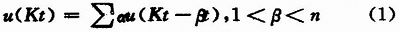这是不断乘累加的过程，解决了滤波器的系数α问题，再加上乘法和加法计算即可实现滤波器设计。由于FIR滤波器的单位脉冲响应h(n)是有限长序列，因此滤波器没有不稳定的问题，FIR滤波器一般为非递归结构，因此在采用Matlab设计时采用有限精度的计算，以避免出现递归结构中极性震荡等不稳定现象。常见的两种FIR滤波器设计方法是窗函数法和频率采样法。虽然频率采样法可以精确控制采样点的频率响应，但是设计中必须插入过渡点来改善纹波，而且截止频率不易控制，过渡点也需要进一步的优化，对比来看窗函数法则是一种基本的设计理念，设计方法比较成熟。并且Matlab中提供的函数可以方便地实现加窗线性相位FIR滤波器设计，包括了比较常见的低通、带通、高通和带阻数字滤波器。本文采用的是窗函数结合编程的设计方法。

窗函数法的基本思想是先给定理想的滤波器频响为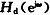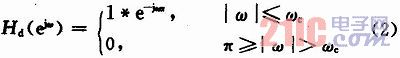式中：ωc为截止频率；α为采样延迟。

而所要求设计的频响为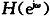，之后的工作便是使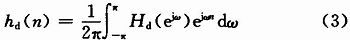逼近

。加窗w(n)对理想滤波器的单位抽样响应hd(n)(见式(3))截断，得到所要设计的h(n)。对于FDATool设计法，本文通过选择适当参数，利用Matlab完成。

2 FIR数字滤波器设计

2．1 窗函数法设计FIR滤波器方案

在Matlab中可直接产生窗函数：矩形窗(Rectangle Window)，三角窗(Triangular Window)，汉宁窗(Hanging Window)，凯塞窗(Kaiser Window)等，通过调用系统的函数即可实现窗的加载。具体调用方法如下：调用格式：w=函数名(n)，根据长度n产生一个矩形窗w。一般正常的心电信号频率范围在0．05～100 Hz之内，这本身就是一种比较微弱的电信号，当受到身体其他器官的干扰信号后，心电信号将会严重失真。另外还要考虑到电子器件噪声和50 Hz的工频信号的存在。这就需要尽量消除噪声和干扰的影响。这里选取低通滤波器的设计指标为：通带截止频率ωp=0．2π，阻带截止频率ωs=0．3π，最小阻带衰减As≥50 dB。所以得出过渡带宽tr_width=ωs-ωp，列长N=10 π／tr_width。选择窗函数一般是选择主瓣较宽的，这样可以增加阻带的衰减，保证了通带的平稳，另外在保证阻带最小衰减指标的情况下，适当增加列长N值，窄化过渡带。根据窗函数最小阻带衰减的特性。只有海明窗和凯塞窗可提供大于50 dB的衰减。实际应用中选用窗函数大多是它们的折中，凯塞窗可以通过改变参数值来折中选择主瓣宽度和旁瓣衰减，基于此的滤波器适应能力强且比较灵活。本文即是采用的凯塞窗编程设计。窗函数设计法是用一定宽度窗函数截取无限脉冲响应序列获得有限长的脉冲响应序列，设计步骤为：

(1)通过傅里叶逆变换获得理想滤波器的单位脉冲响应hd(n)。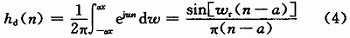(2)由性能指标确定窗函数W(n)和窗口长度N。

(3)求得实际滤波器的单位脉冲响应h(n)，h(n)即为所设计FIR滤波器系数向量a(n)。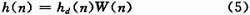(4)检验滤波器性能。

Matlab信号处理工具箱提供了各种窗函数、滤波器设计函数和滤波器实现函数。设计中利用Matlab提供的函数firl来实现，编程则是直接调用这些函数简单直观的完成设计。调用格式：firl(n，Wn，‘ftype’，Window)，其中n为阶数、Wn为截止频率、ftype是滤波器的类型、Window是窗函数。应用凯塞窗的代码如下：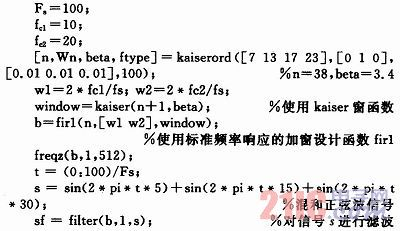程序执行的结果如图1，图2所示。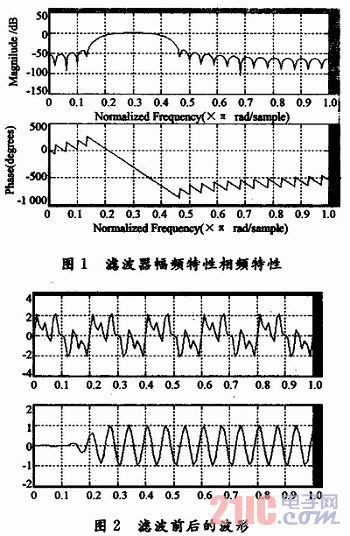从滤波效果图看，所设计的滤波器基本消除了噪声和干扰的影响，客观的得出真实信号。按照设计方案中滤波器的指标，执行函数操作可以得到：N=68，As=59。之后增加参数N值。在该设计中，利用凯塞窗函数，执行了多种N值的改变。伴随N值增大，过渡带变窄，但阻带的最小衰减没有改变。

2．2 FDATool设计方案

FDATool(Filter Design＆Analysis Too1)是Matlab信号处理工具箱专用的滤波器设计分析工具，操作简单、灵活，可以采用多种方法设计FIR滤波器。这种方法设计的数字滤波器，可以随时调整滤波器滤披特性，而且滤波结果实时显示在图形区，减少了工作量，有利于滤波器设计的进一步优化。在Matlab命令窗口输入FDATool后回车会弹出FDATool界面，也可在Matlab主界面下方选择“start”→“toolbox”→“ filterdesign”。具体参数选择为：

(1)滤波器类型(filter type)为lowpass；

(2)设计方法(design method)为FIR，使用窗口Window；

(3)滤波器的阶数(fiter order)为15；

(4)窗口类型(Window)为Kaiser，beta为0．5；

(5)fs为48 000 Hz，fc为10 800 Hz。

点击“design filter”即可。值得注意的是，这里选择filter order为15阶，而不是所设计的16阶滤波器。因为常数系数项h(0)=0。通过菜单“analysis”选择“magnitude”和“phase response”即可得到图3，图4的特性显示。“analysis”选择“step response”和“im-pulse”可以得到图5，图6的响应显示。可以看出所设计FIR滤波器的阶跃响应和冲击响应比较接近理想状态。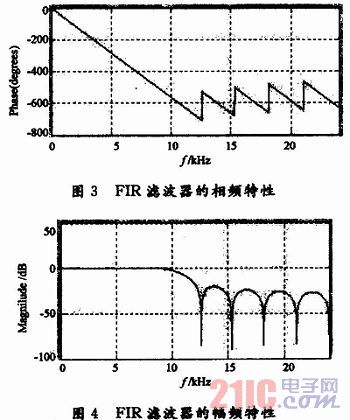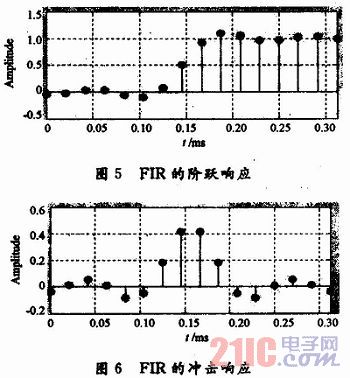3 结论

从图1～4的特性曲线中可以看出该滤波器的性能基本达到了妻求，滤波作用比较明显。曲线相对比较平稳，能够满足微弱信号滤波器的设计技术指标。特别是从方案二中的相频特性曲线来看，曲线通过原点且为一条平滑直线，说明具有良好的线性相位特性，而这也是在应用中选择FIR数字滤波器的重要原因，但是它的实际效果还要经过实践的检验。FIR幅频特性精度比IIR低，且滤波器所需阶次比较高，但是它拥有很好的线性相位，即不同频率分量的信号经过FIR滤波器后他们的时问差不变。另外，FIR还存在的缺点应加以改善，如信号的延迟偏大。这就考虑到用高性能的DSP器件，由于其处理速度快，此缺点在一点程度上是可以改善的。

4 结语

本文通过设计实例，介绍了利用Matlab实现数字信号处理中的数字滤波器设计，从结果可以看出它们均可以达到技术指标要求，而且方法简单、快捷，大大减轻了工作量。滤波器的设计工作完成后，可以借助Matlah的export操作导出所设计滤波器的系统函数H(x)。由于Matl-ah具有强大的接口功能，仿真后的结果可以很方便的移植到DSP，CPLD或FPGA等器件中。在实际应用中，只需按要求修改滤波器参数，并对程序作较少的改动，即可实现不同的滤波器，实用性较强。

展开全文• 目录：MATLAB-FIR数字滤波器设计1 FIR滤波器的原理2 FIR滤波器的特点2.1 相位特性2.1.1 偶对称2.1.2 奇对称2.2 幅度特性3 几种滤波器函数3.1 fir1()3.2 fir2()3.3 kaiserord()3.4 firpm()4 filterDesigner配置4.1 ...

### 目录：

本文是基于MATLAB的数字滤波器设计，所有数据基于计算机内部处理，因而都是离散信号，所以采用的是数字滤波器。从实现的网络结构或者单位脉冲响应来看，数字滤波器可以分为FIR(Finite Impulse Response , 有限脉冲响应)滤波器和IIR(Infinite Impulse Response , 无线脉冲响应)滤波器。本文主要从两个方面介绍FIR滤波器的MATLAB设计：集成函数和工具箱。

## 1 FIR滤波器的原理

从时域看，FIR的一般表达式H(z)如下：

∑ n = 0 N − 1 h ( n ) z − n = h ( 0 ) + h ( 1 ) z − 1 + . . . + h ( N − 1 ) z − ( N − 1 ) \sum_{n=0}^{N-1}h(n)z^{-n}=h(0)+h(1)z^{-1}+...+h(N-1)z^{-(N-1)}

从表达式至少可以看出两点：系统只在原点处有极点，可以用抽头法构建模型，每一个乘法器的系数即为抽头系数。
由于N是有限值，因此抽头系数项是有限的，可以得到h(n)：

∑ n = 0 N − 1 h ( 0 ) δ ( n ) + h ( 1 ) δ ( n − 1 ) + . . . + h ( N − 1 ) δ ( n − ( N − 1 ) ) \sum_{n=0}^{N-1}h(0)δ(n)+h(1)δ(n-1)+...+h(N-1)δ(n-(N-1))

可以看出h(n)，即系统的脉冲响应的项数是有限的，因此成为有限脉冲响应。

## 2 FIR滤波器的特点

FIR一个突出的优点就是具有严格的线性相位特性，但并不是所有结构的FIR都具备此特性，只有当FIR滤波器的单位脉冲响应满足对称条件时，FIR才具有线性相位特性。

### 2.1 相位特性

研究FIR的相位特性时，将结构分为奇对称和偶对称两种。

#### 2.1.1 偶对称

当FIR单位脉冲响应满足偶对称，即有：

h ( n ) = h ( M − n ) , 0 ≤ n ≤ M h(n)=h(M-n), 0 ≤ n ≤ M

代入H(z)的表达式：

∑ n = 0 M h ( n ) z − n = ∑ n = 0 M h ( M − n ) z − n \sum_{n=0}^{M}h(n)z^{-n}=\sum_{n=0}^{M}h(M-n)z^{-n}

令M-n=k , 对等号右边部分做变换：

H ( z ) = ∑ k = 0 M h ( k ) z − ( M − k ) = z − M ∑ n = 0 M h ( k ) z k = z − M H ( z − 1 ) H(z)=\sum_{k=0}^{M}h(k)z^{-(M-k)}=z^{-M}\sum_{n=0}^{M}h(k)z^k=z^{-M}H(z^{-1})

所以H(z)有两种表达方式，那么H(z)可以表示为：

H ( z ) = 1 2 [ H ( z ) + z − M H ( z ) ] = 1 2 ∑ k = 0 M h ( n ) [ z − n + z − M z n ] H(z)=\frac{1}{2}[H(z)+z^{-M}H(z)]=\frac{1}{2}\sum_{k=0}^{M}h(n)[z^{-n}+z^{-M}z^{n}]

提取一项 z − M 2 z^{-\frac{M}{2}} ，可得到滤波器频响：

H ( e j w ) = e − j w M 2 ∑ n = 0 M h ( n ) c o s [ w ( M 2 − n ) ] = A ( w ) e − j w M 2 H(e^{jw})=e^{-jw\frac{M}{2}}\sum_{n=0}^{M}h(n)cos[w(\frac{M}{2}-n)]=A(w)e^{-jw\frac{M}{2}}

根据相频定义，可知FIR相频为：

φ ( w ) = − M 2 w φ(w)=-\frac{M}{2}w

故满足线性相位特性。

#### 2.1.2 奇对称

当FIR单位脉冲响应满足偶对称，即有：

h ( n ) = − h ( n − M ) , 0 ≤ n ≤ M h(n)=-h(n-M), 0 ≤ n ≤ M

由2.1.1推导思路可得：

H ( e j w ) = e − j w M 2 + π 2 ∑ n = 0 M h ( n ) s i n [ w ( M 2 − n ) ] = A ( w ) e − j w M 2 + π 2 H(e^{jw})=e^{-jw\frac{M}{2}+\frac{π}{2}}\sum_{n=0}^{M}h(n)sin[w(\frac{M}{2}-n)]=A(w)e^{-jw\frac{M}{2}+\frac{π}{2}}

根据相频定义，可知FIR相频为：

φ ( w ) = − M 2 w + π 2 φ(w)=-\frac{M}{2}w+\frac{π}{2}

故满足线性相位特性。

### 2.2 幅度特性

研究FIR的幅度特性时，将结构分别分为偶数和奇数的偶对称和奇对称四种。推导方式和相位特性类似，这里直接给出结论。

单位脉冲响应特征相位特性幅度特性滤波器种类
偶对称，偶整数线性相位对于w=0，π ，2π为偶对称适合各种滤波器
偶对称，奇整数线性相位对于w=π为奇对称，对于w=0、2π为偶对称，w=π处为0不适合高通，带阻
奇对称，偶整数线性相位，附加90°相移对于w=0、π、2π均为奇对称，在w=0、π、2π处都为0只适合带通
偶对称，奇整数线性相位，附加90°相移对于w=0、2π均为奇对称，在w=π处为偶对称，在w=0、2π处为0适合高通、带通

## 3 几种滤波器函数

### 3.1 fir1()

• fir1函数语法形式
b=fir1(n,wn,'ftype',window,'noscale');
% n     滤波器阶数
% wn    类型和意义与ftype有关，wn的取值范围为(0,1)，1代表fs的1/2。
% 当wn为单值，表示截止频率。若ftype为'low'，表示低通；若ftype为'high'，表示高通。
% 当wn为[wn1 wn2]，即两个元素组成的向量，则表示带通或带阻，ftype对应'bandpass'或'stop'。
% 当wn由多个数组成的向量[w1 w2...wn]，其中w1<w2<…<wn，则fir1返回带0<ω<w1，w1<ω<w2，…，wn<ω<1的n阶多带滤波器。
% ftype 滤波器类型
% 'low'       -低通
% 'high'      -高通
% 'bandpass'  -带通
% 'stop'      -带阻
% 'DC-0'      -第一带为带阻
% 'DC-1'	  -第一带为带通
% window  默认为海明窗(Hamming),还有汉宁窗(Hanning),布拉克曼窗(Blackman)和凯塞窗(Kaiser)
% noscale 指定归一化滤波器幅度


### 3.2 fir2()

fir2在fir1的基础上，还可以指定频段内的理想幅值，即任意响应滤波器。

• fir2函数语法形式
b=fir2(n,fm,m,npt,lap,window);
% n        滤波器阶数
% f和m     f是一个频段向量，在(0,1)内分布,对应归一化频率;m是对应频段理想幅值
% npt      正整数，指定对幅频进行插值的点数，默认为512
% lap      插值时，将非连续点转化成连续点的点数，默认为25
% window   窗函数，默认为海明窗


### 3.3 kaiserord()

凯塞窗，根据一些期望的参数，得到设计滤波器所需要的参数，可以用于设计最优滤波器。

• kaiserord函数语法形式
[n,wn,beta,ftype]=kaiserord(f,a,dev,fs);
% 输入
% f   偶数个元素的向量，表示过渡带的起点到终点，过渡带的增益。
% eg:[1200 1300 1500 1600]表示过渡带1200~1300Hz,1500~1600Hz。fs为采样频率。如果没有fs，f元素取值在(0,1)，即自动归一化;如果有fs，f就为实际频率。
% a   f确定了过渡带，a确定各频段的理想幅值。通带设置为1，阻带设置为0。
% 假设f=[0 0.3 0.4 0.6 0.7 1.0] , a=[0 1 0 0 0.5 0.5]
% dev 向量，各频段纹波。
% 输出
% n     返回滤波器阶数
% wn    向量，返回滤波器截止频率点
% beta  返回凯塞窗的beta值
% ftype 返回滤波器类型### 3.4 firpm()

既能设计任意响应，又能设计最优滤波器，还能附加90°相移

• firpm函数语法形式
[b,delta]=firpm(n,f,a,w,'ftype');
% n,f,a与kaiserord函数一样
% w,对应频段幅值的权值,权值越高，越接近理想状态
% ftype 滤波器结构。默认为偶对称脉冲响应，'hilbert'为奇对称结构，即具有90°相移特性
% delta 最大纹波


## 4 仿真实例

需求：利用凯塞窗设计一个低通FIR滤波器，过渡带为1000~1500Hz，采样频率为8000Hz，通带纹波最大为0.01，阻带纹波最大为0.05。利用海明窗及firpm函数设计相同的低通滤波器，截止频率为1500Hz，滤波器阶数为凯赛窗函数求取的值。绘出三种方法设计的幅度频率响应曲线。

### 4.1 代码


%% 低通；过渡带为1~1.5KHz，采样频率8KHz，通带最大纹波0.01，阻带最大纹波0.05

% 获取凯塞窗参数,后续fir1要设计凯赛窗的低通滤波器
fs=8000;                                   % 采样频率
fc=[1000 1500];                            % 过渡带；1000~1500过渡带.通带:0~(1000*2/fs),阻带:(1500*2/fs)~1
mag=[1 0];                                 % 理想幅值.0~1000*2/fs是1，1500*2/fs~1是0
dev=[0.01 0.05];                           % 纹波
[n,wn,beta,ftype]=kaiserord(fc,mag,dev,fs);% 获取凯塞窗参数,根据过渡带幅值返回ftype类型

% fir1设计凯塞窗和海明窗滤波器
h_kaiser=fir1(n,wn,ftype,kaiser(n+1,beta));
h_hamm=fir1(n,fc(2)*2/fs);                 %fc(2)=1500Hz,LPF截止频率

% 设计最优滤波器
fpm=[0 fc(1)*2/fs fc(2)*2/fs 1];           % firpm频段向量,归一化[0 1]
magpm=[1 1 0 0];% firpm幅值向量
h_pm=firpm(n,fpm,magpm);                   %设计低通滤波器

% 幅频
m_kaiser=20*log(abs(fft(h_kaiser,1024)))/log(10);
m_hamm=20*log(abs(fft(h_hamm,1024)))/log(10);
m_pm=20*log(abs(fft(h_pm,1024)));

% 设置幅频响应的横坐标为Hz
x_f=[0:1:length(m_kaiser)/2]*fs/length(m_kaiser);

% 只显示正频率部分
m1=m_kaiser(1:length(x_f));
m2=m_hamm(1:length(x_f));
m3=m_pm(1:length(x_f));

% 绘制幅频曲线
plot(x_f,m1,'-',x_f,m2,'-.',x_f,m3,'--');
xlabel('频率(Hz)');
ylabel('幅度(dB)');
legend('凯塞窗','海明窗','最优滤波器');
grid;



### 4.2 仿真分析

如图所示，绘制了三种滤波器。如果要用凯塞窗设计fir1，那么先要利用kaiserord函数获取凯塞窗的相关参数，即第一种滤波器。如果设计海明窗，则输入截止频率即可(要归一化后的频率，否则需要输入采样频率)。设计最优滤波器输入频段和相应幅度即可，这里需要和前两种做比较，因此都是输入n阶。展开全文• 西 南 石 油 大 学 实 验 报 告 课 程 数字信号处理 实验项目 利用MATLAB进行基于窗函数的FIR数字滤波器设计 成 绩 专业年级 通信工程09 学 号 0907050228 指导教师 姓 名 王延礼 同组人姓名 实验日期 一 实验目的...
• 随着计算机和信息技术的快速发展，数字信号处理技术在通信与电子技 术、航空航天、仪器仪表等诸多领域得到了...用软件来实现数字滤波器的优点是随着滤波器参数的改变，很容易改变滤波器性能，从而满足设计需求。
• 1.实验目的 （1）掌握用窗函数法设计 FIR 数字滤波器的原理和方法。 （2）熟悉线性相位 FIR 数字滤波器特性。 （3）了解各种窗函数对滤波特性的影响。
• 摘 要 :阐迷了FIR数字浦波器的窗a数设计方法，并利用Matlab语言实现了浦波器的设计与仿真。Matlab语言可以方便、快捷地设计具有严格线性相位的FIR系跳，可节省大It的编程时间，提高编租效率，且参数的修改也十分方便...

摘 要 :阐迷了FIR数字浦波器的窗a数设计方法，并利用Matlab语言实现了浦波器的设计与仿真。Matlab语言可以

方便、快捷地设计具有严格线性相位的FIR系跳，可节省大It的编程时间，提高编租效率，且参数的修改也十分方便。随着

版本的不断提高，Matlab在数字滤渡技术中会发挥更大的作用。

关 健 询 :FIR;数字滤波器;窗a数;Matlab

中 图 分 类号:TN911 文献标识码:B 文童偏号:1004一373X(2007)17一047一02

De sig n o fM atlabB asedo nF IR DigitalF ilter

RU X ia oy an ,S H IL in g

(COlleze d Enzineerina Technolopy,Yunnen A.6-11.i Uni- itr,Kunmi一650201,Chi..)

Abs tra ct :Th isp aperel aboratest heF IR digitalfi ltersw indowsf unctiond esignm ethod,an dr ealizest hef ilterd esigna nd

simulation byu singt heM atlabl anguage.T hisL anguagec and esignt hes trictl inearp haseF IR system convenientlya ndq uickly,

al soi tc ans avem anyp rogrammingt ime,en hancest hep rogramminge fficiency,an dt hep arameterr evisioni sa lsoc onvenient.

We believe that the Matlab language can play an important role in the digital filter technology with the improvement of edition

enhancement unceasingly.

Ke yw or ds:F IR;digitalf ilter;w indowsf unction;M atlab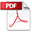2008-5-9 00:08 上传

点击文件名下载附件

133.39 KB, 下载次数: 34446

基于FIR数字滤波器的Matlab设计

展开全文...

# fir数字滤波器matlab设计matlab 订阅